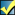A Flash Developer Resource Site

# Thread: [RESOLVED] Bubbles/Ball Mousover Random

1. ##[RESOLVED] Bubbles/Ball Mousover Random

Ok, so below is a code I found and modified to create some bubbles floating around in the background on a site. There is one issue though, the client wans the bubbles to pop into different colored logos. I figured the best way to do this would to have it change to a random logo upon a mouse over (so they wouldn't all pop at once). the problem is I can't seem to get this to recognize a mouse over and change. You can see my attempt to get it to work in the code, but if you have any alternative ideas I would really appreciate it.

The ball is made of a 5 frame movie clip symbol. The first frame is the bubble and the other 4 are the logos.

If this is just not going to be possible no mater what I try please let me know.

Thanks.

Code:
```kNbrBalls = 20;
gravK = -0.0;			// gravity
dampK = 01;       	// lower numbers make more air resistance
dampCollision = .99; 	// energy retained in collisions

SH = Stage.height;
SW = Stage.width;

MovieClip.prototype.handleCollisions = function()
{
for (var i = mcs.length-1; i >= 1; --i) {
var x1 = mcs[i]._x;
var y1 = mcs[i]._y;
for (j = i-1; j >= 0; --j) {
var dx = mcs[j]._x - x1;
var dy = mcs[j]._y - y1;
var dist = Math.sqrt(dx*dx+dy*dy);
if (dist < ballWidth) {
// collide - trade energy (mag1,mag2), and travel in opposite direction of collision
var mag1 = Math.sqrt(mcs[i].vx*mcs[i].vx+mcs[i].vy*mcs[i].vy);
var mag2 = Math.sqrt(mcs[j].vx*mcs[j].vx+mcs[j].vy*mcs[j].vy);
mcs[j].vx = (mag1*dx/dist)*dampCollision;
mcs[j].vy = (mag1*dy/dist)*dampCollision;
mcs[i].vx = -(mag2*dx/dist)*dampCollision;
mcs[i].vy = -(mag2*dy/dist)*dampCollision;
}
}
}
}

MovieClip.prototype.moveBall = function()
{
// compute forces on ball
if (!this.isDragging) {
this.vx *= dampK;	// damping due to air resistance
this.vy *= dampK;

this.vy += gravK;	// gravity

// bounce off floor and ceiling
if (this._y+ballRadius + this.vy >= SH ||
(this._y-ballRadius) + this.vy < 0)
{
this.vy *= -dampCollision;
}
// bounce off right and left walls
if ((this._x-ballRadius) + this.vx <= 0 ||
this._x+ballRadius + this.vx >= SW)
{
this.vx *= -dampCollision;
}
this._x += this.vx;
this._y += this.vy;
}
else {
// Set vx,vy to correspond to mouse movement, enabling user to 'toss' ball
this.vx *= dampK;
this.vy *= dampK;
this.vx = _root._xmouse - this.lmx;
this.vy = _root._ymouse - this.lmy;
this.lmx = _root._xmouse;
this.lmy = _root._ymouse;
}
}

mcs = [];
for (var i = 0; i < kNbrBalls; ++i) {
var mc = this.attachMovie("superball_mc", "mc"+i, 1+i);
mcs[i] = mc;
mc.vx = Math.random()*32-16;
mc.vy = Math.random()*32-16;
mc.onEnterFrame = mc.moveBall;
mc.gotoAndStop(1)
mc.cursor.onRollOver=function(){
stop();
choice = Math.round(Math.random()*4);

switch (choice) {

case 0 :

mc.gotoAndStop(1);

break;

case 1 :

mc.gotoAndStop(2);

break;

case 2 :

mc.gotoAndStop(3);

break;

case 3 :

mc.gotoAndStop(4);

break;

case 4 :

mc.gotoAndStop(5);

break;
}
}
}

_root.onEnterFrame = handleCollisions;```Reply With Quote

2. Hi,

I removed the "cursor" from "mc.cursor.onRollOver=function()" at this end as I'm not sure what or where it is, but I'm sure it can be reinstated within the code, perhaps this might get you somewhere:
PHP Code:
``` kNbrBalls = 20; gravK = -0.0;// gravity dampK = 01;// lower numbers make more air resistance dampCollision = .99;// energy retained in collisions ballRadius = 15; ballWidth = ballRadius * 2; SH = Stage.height; SW = Stage.width; MovieClip.prototype.handleCollisions = function() {     for (var i = mcs.length - 1; i >= 1; --i)     {         var x1 = mcs[i]._x;         var y1 = mcs[i]._y;         for (j = i - 1; j >= 0; --j)         {             var dx = mcs[j]._x - x1;             var dy = mcs[j]._y - y1;             var dist = Math.sqrt(dx * dx + dy * dy);             if (dist < ballWidth)             {                 // collide - trade energy (mag1,mag2), and travel in opposite direction of collision                 var mag1 = Math.sqrt(mcs[i].vx * mcs[i].vx + mcs[i].vy * mcs[i].vy);                 var mag2 = Math.sqrt(mcs[j].vx * mcs[j].vx + mcs[j].vy * mcs[j].vy);                 mcs[j].vx = (mag1 * dx / dist) * dampCollision;                 mcs[j].vy = (mag1 * dy / dist) * dampCollision;                 mcs[i].vx = -(mag2 * dx / dist) * dampCollision;                 mcs[i].vy = -(mag2 * dy / dist) * dampCollision;             }         }     } }; MovieClip.prototype.moveBall = function() {     // compute forces on ball     if (!this.isDragging)     {         this.vx *= dampK;// damping due to air resistance         this.vy *= dampK;         this.vy += gravK;// gravity         // bounce off floor and ceiling         if (this._y + ballRadius + this.vy >= SH || (this._y - ballRadius) + this.vy < 0)         {             this.vy *= -dampCollision;         }         // bounce off right and left walls            if ((this._x - ballRadius) + this.vx <= 0 || this._x + ballRadius + this.vx >= SW)         {             this.vx *= -dampCollision;         }         this._x += this.vx;         this._y += this.vy;     }     else     {         // Set vx,vy to correspond to mouse movement, enabling user to 'toss' ball         this.vx *= dampK;         this.vy *= dampK;         this.vx = _root._xmouse - this.lmx;         this.vy = _root._ymouse - this.lmy;         this.lmx = _root._xmouse;         this.lmy = _root._ymouse;     } }; mcs = []; for (var i = 0; i < kNbrBalls; ++i) {     var mc = this.attachMovie("superball_mc", "mc" + i, 1 + i);     mcs[i] = mc;     mc._x = ballRadius + random(Stage.width - ballWidth);     mc._y = ballRadius + random(Stage.height - ballWidth);     mc.vx = Math.random() * 32 - 16;     mc.vy = Math.random() * 32 - 16;     mc._width = ballRadius * 2;     mc._height = ballRadius * 2;     mc.onEnterFrame = mc.moveBall;     mc.gotoAndStop(1);     mc.onRollOver = function()     {         delete this.onEnterFrame;         choice = Math.round(1 + (4 - 1) * Math.random());         trace(choice + " : " + this);         switch (choice)         {                 //case 0 :                 //this.gotoAndStop(1);                 //break;             case 1 :                 this.gotoAndStop(2);                 break;             case 2 :                 this.gotoAndStop(3);                 break;             case 3 :                 mc.gotoAndStop(4);                 break;             case 4 :                 this.gotoAndStop(5);                 break;         }     };     mc.onRollOut = function()     {         this.onEnterFrame = this.moveBall;         this.gotoAndStop(1);     }; } _root.onEnterFrame = handleCollisions;  ```
alternativelt, attach your flaReply With Quote

3. Thanks for the help fruitbeard. I actually just figured out a way to randomize it that works without a mouseover. However now I have a new problem with this I discovered after trying to implement it: http://board.flashkit.com/board/show...21#post4317321Reply With Quote

#### Tags for this Thread

as2, bouncing balls, mouse over, movie clip symbol, random####Posting Permissions

• You may not post new threads
• You may not post replies
• You may not post attachments
• You may not edit your posts
•

 » Home » Movies » Tutorials » Submissions » Board » Links » Reviews » Feedback » Gallery » Fonts » The Lounge » Sound Loops » Sound FX » About FK » Sitemap

Click Here to Expand Forum to Full Width